# 聊天机器人（chatbot）终极指南：自然语言处理（NLP）和深度机器学习（Deep Machine Learning）

## 概述：聊天机器人开发中的深度学习技术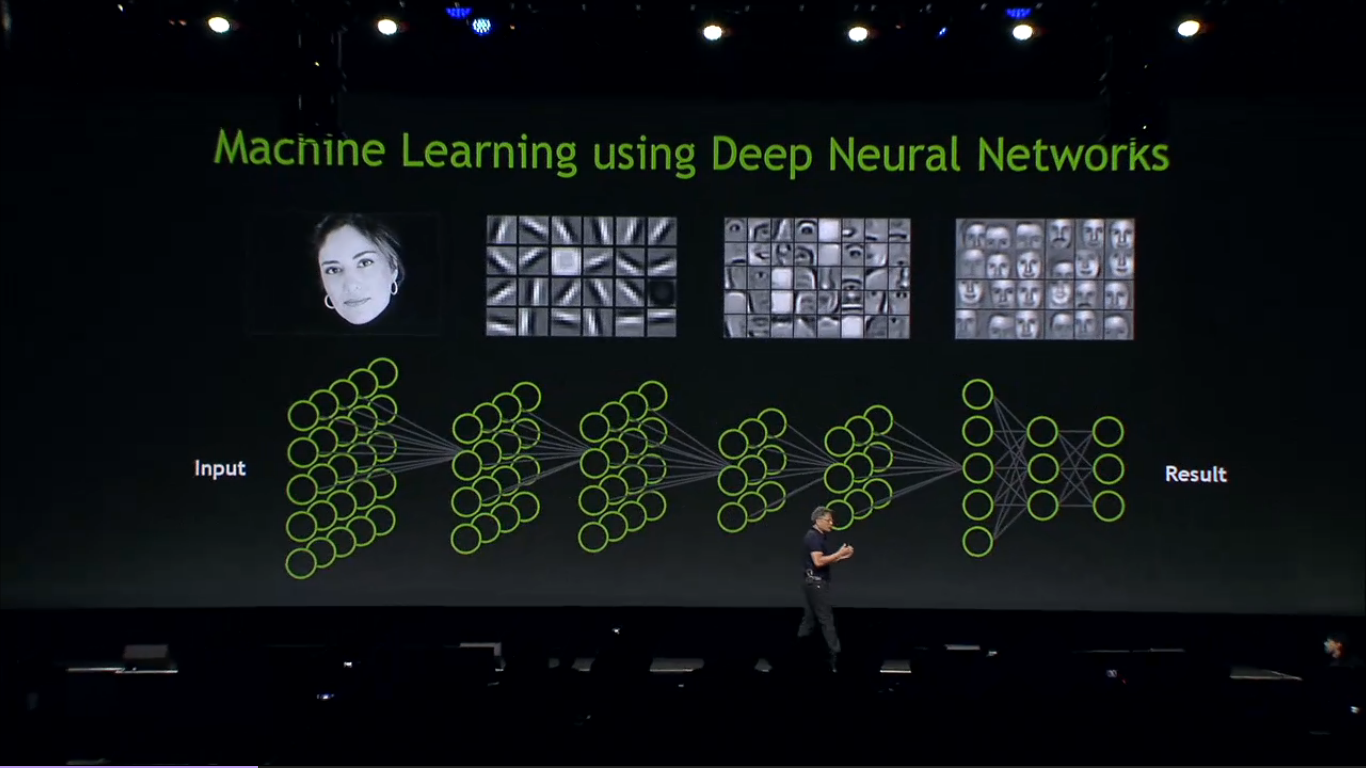## 模型分类### 基于检索的模型 VS. 生成式模型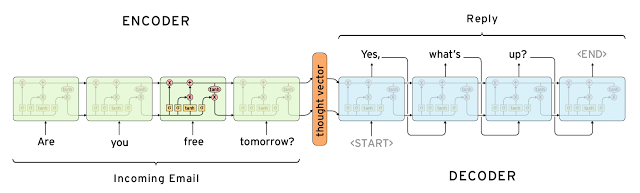### 对话的长短### 开放领域 VS. 封闭领域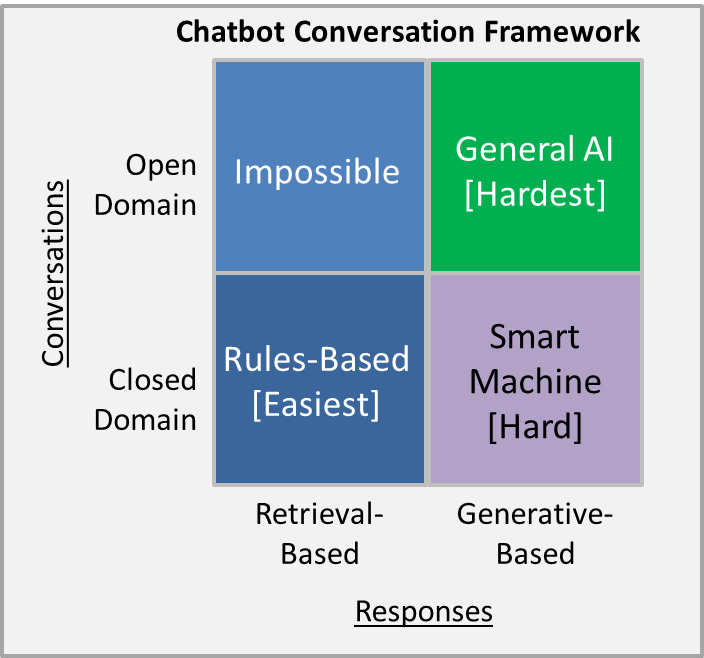“开放领域 ：可以提出一个关于任何主题的问题，并期待相关的回应，这很难实现。考虑一下，如果就抵押贷款再融资问题进行交谈的话，实际上你可以问任何事情“ —— 马克·克拉克

“封闭领域 ：可以问一些关于特定主题的有限的问题，更容易实现。比如，迈阿密天气怎么样？“

“Square 1迈出了一个聊天机器人的可喜的第一步，它表明了可能不需要智能机器的复杂性，也可以提供商业和用户价值。

”Square 2使用了可以生成响应的智能机器技术。 生成的响应允许Chatbot处理常见问题和一些不可预见的情况，而这些情况没有预定义的响应。 智能机器可以处理更长的对话并且看起来更像人。 但是生成式响应增加了系统的复杂性，而且往往是增加了很多的复杂性。

## 共同的挑战

### 使用上下文信息### 一致的个性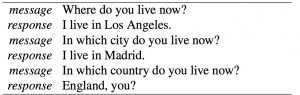### 模型的评估## 现在能实现到什么程度？

（前）百度首席科学家Andrew Ng 最近接受采访时说：

## 用TENSORFLOW实现一个基于检索的模型

### UBUNTU DIALOG CORPUS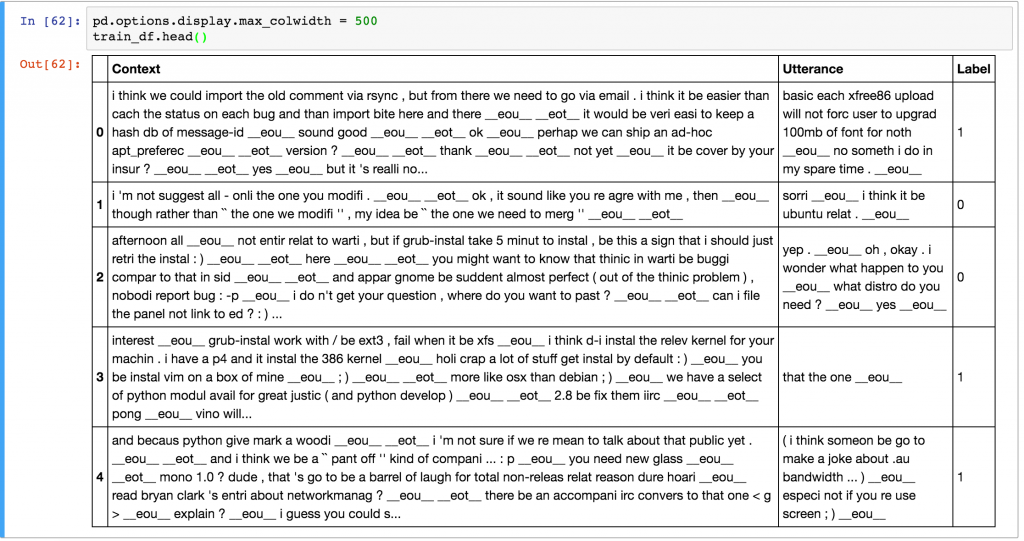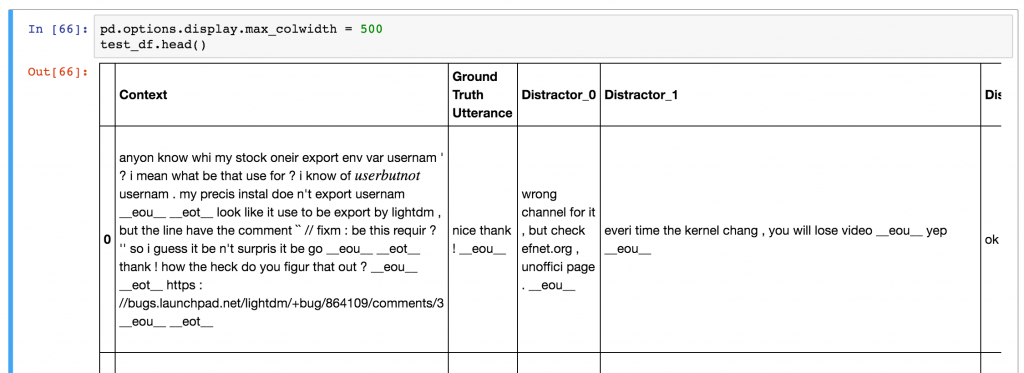### 基准

def evaluate_recall(y, y_test, k=1):
num_examples = float(len(y))
num_correct = 0
for predictions, label in zip(y, y_test):
if label in predictions[:k]:
num_correct += 1
return num_correct/num_examples
1234567

# Random Predictor
def predict_random(context, utterances):
return np.random.choice(len(utterances), 10, replace=False)

# Evaluate Random predictor
y_random = [predict_random(test_df.Context[x], test_df.iloc[x,1:].values) for x in range(len(test_df))]

y_test = np.zeros(len(y_random))
for n in [1, 2, 5, 10]:
print(“Recall @ ({}, 10): {:g}”.format(n, evaluate_recall(y_random, y_test, n)))
12345678910

Recall @ (1, 10): 0.0937632
Recall @ (2, 10): 0.194503
Recall @ (5, 10): 0.49297
Recall @ (10, 10): 1
1234

class TFIDFPredictor:
def __init__(self):
self.vectorizer = TfidfVectorizer()

def train(self, data):
self.vectorizer.fit(np.append(data.Context.values,
data.Utterance.values))
def predict(self, context, utterances):
# Convert context and utterances into tfidf vector
vector_context = self.vectorizer.transform([context])
vector_doc = self.vectorizer.transform(utterances)

# The dot product measures the similarity of the resulting vectors
result = np.dot(vector_doc, vector_context.T).todense()
result = np.asarray(result).flatten()

# Sort by top results and return the indices in descending order
return np.argsort(result, axis=0)[::-1]

# Evaluate TFIDF predictor
pred = TFIDFPredictor()
pred.train(train_df)

y = [pred.predict(test_df.Context[x], test_df.iloc[x,1:].values) for x in range(len(test_df))]

for n in [1, 2, 5, 10]:
print(“Recall @ ({}, 10): {:g}”.format(n, evaluate_recall(y, y_test, n)))
12345678910111213141516171819202122232425262728

Recall @ (1, 10): 0.495032

Recall @ (2, 10): 0.596882

Recall @ (5, 10): 0.766121

Recall @ (10, 10): 1
1234567

### 双编码器LSTM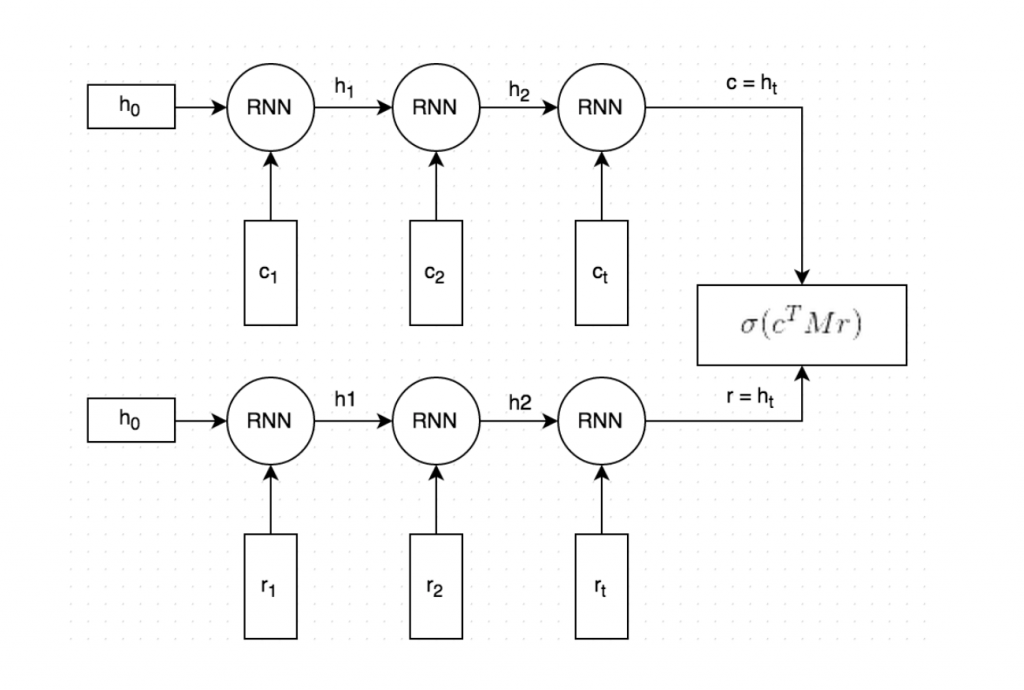1. 上下文和响应文本都是按照单词分割的，每个单词都嵌入到一个向量中。 词嵌入是用斯坦福大学的GloVe矢量进行初始化的，并且在训练过程中进行了微调（注：这是可选的，并且没有在图片中显示，我发现用GloVe进行初始化对模型性能没有太大的影响）。
2. 嵌入的上下文和响应都逐字地输入到相同的递归神经网络（Recurrent Neural Network）中。 RNN生成一个矢量表示，不严格地说，这个表示捕捉了上下文和响应（图片中的c和r）中的“含义”。 我们可以自由选择矢量的大小，不过先选择256个维度吧。
3. 我们用矩阵M乘以c来“预测”一个响应r'。 如果c是256维向量，则M是256×256维矩阵，结果是另一个256维向量，我们可以将其解释为产生的响应。 矩阵M是在训练中学习到的。
4. 我们通过取这两个向量的点积来度量预测响应r'和实际响应r的相似度。 大的点积意味着两个向量更相似，因此应该得到高分。 然后，我们应用sigmoid函数将该分数转换为概率。 请注意，步骤3和4在图中组合在一起。

### 数据预处理

• context：表示上下文文本的词序列，例如[231,2190,737,0,912]
• context_len：上下文的长度，例如上面例子中的5
• utterance：表示话语（响应）的一系列单词id
• utterance_len：话语的长度
• label：标签，在训练数据中才有。 0或1。
• distractor_ [N]：仅在测试/验证数据中。 N的范围从0到8.代表干扰项的词序列id。
• distractor_ [N] _len：仅在测试/验证数据中。 N的范围是从0到8.发音的长度。

### 创建一个输入函数

def input_fn():
# TODO Load and preprocess data here
return batched_features, labels
123

def create_input_fn(mode, input_files, batch_size, num_epochs=None):
def input_fn():
# TODO Load and preprocess data here
return batched_features, labels

return input_fn
123456

1. 创建描述样例文件中字段的特征定义（feature definition
2. 使用tf.TFRecordReader从输入文件中读取记录
3. 根据特征定义解析记录
4. 提取训练标签
5. 将多个样例和培训标签构造成一个批次
6. 返回批次

### 定义评估指标

def create_evaluation_metrics():
eval_metrics = {}
for k in [1, 2, 5, 10]:
eval_metrics[“recall_at_%d” % k] = functools.partial(
tf.contrib.metrics.streaming_sparse_recall_at_k,
k=k)
return eval_metrics
1234567### 训练代码样板

estimator = tf.contrib.learn.Estimator(
model_fn=model_fn,
model_dir=MODEL_DIR,
config=tf.contrib.learn.RunConfig())

input_fn_train = udc_inputs.create_input_fn(
mode=tf.contrib.learn.ModeKeys.TRAIN,
input_files=[TRAIN_FILE],
batch_size=hparams.batch_size)

input_fn_eval = udc_inputs.create_input_fn(
mode=tf.contrib.learn.ModeKeys.EVAL,
input_files=[VALIDATION_FILE],
batch_size=hparams.eval_batch_size,
num_epochs=1)

eval_metrics = udc_metrics.create_evaluation_metrics()

# We need to subclass theis manually for now. The next TF version will
# have support ValidationMonitors with metrics built-in.
# It’s already on the master branch.
class EvaluationMonitor(tf.contrib.learn.monitors.EveryN):
def every_n_step_end(self, step, outputs):
self._estimator.evaluate(
input_fn=input_fn_eval,
metrics=eval_metrics,
steps=None)

eval_monitor = EvaluationMonitor(every_n_steps=FLAGS.eval_every)
estimator.fit(input_fn=input_fn_train, steps=None, monitors=[eval_monitor])
123456789101112131415161718192021222324252627282930

### 创建模型

def dual_encoder_model(
hparams,
mode,
context,
context_len,
utterance,
utterance_len,
targets):

# Initialize embedidngs randomly or with pre-trained vectors if available
embeddings_W = get_embeddings(hparams)

# Embed the context and the utterance
context_embedded = tf.nn.embedding_lookup(
embeddings_W, context, name=”embed_context”)

utterance_embedded = tf.nn.embedding_lookup(
embeddings_W, utterance, name=”embed_utterance”)

# Build the RNN
with tf.variable_scope(“rnn”) as vs:
# We use an LSTM Cell
cell = tf.nn.rnn_cell.LSTMCell(
hparams.rnn_dim,
forget_bias=2.0,
use_peepholes=True,
state_is_tuple=True)

# Run the utterance and context through the RNN
rnn_outputs, rnn_states = tf.nn.dynamic_rnn(
cell,
tf.concat(0, [context_embedded, utterance_embedded]),
sequence_length=tf.concat(0, [context_len, utterance_len]),
dtype=tf.float32)

encoding_context, encoding_utterance = tf.split(0, 2, rnn_states.h)

with tf.variable_scope(“prediction”) as vs:
M = tf.get_variable(“M”,
shape=[hparams.rnn_dim, hparams.rnn_dim],
initializer=tf.truncated_normal_initializer())

# “Predict” a response: c * M
generated_response = tf.matmul(encoding_context, M)
generated_response = tf.expand_dims(generated_response, 2)
encoding_utterance = tf.expand_dims(encoding_utterance, 2)

# Dot product between generated response and actual response
# (c * M) * r
logits = tf.batch_matmul(generated_response, encoding_utterance, True)
logits = tf.squeeze(logits, )

# Apply sigmoid to convert logits to probabilities
probs = tf.sigmoid(logits)

# Calculate the binary cross-entropy loss
losses = tf.nn.sigmoid_cross_entropy_with_logits(logits, tf.to_float(targets))

# Mean loss across the batch of examples
mean_loss = tf.reduce_mean(losses, name=”mean_loss”)

return probs, mean_loss
1234567891011121314151617181920212223242526272829303132333435363738394041424344454647484950515253545556575859606162

model_fn = udc_model.create_model_fn(
hparams=hparams,
model_impl=dual_encoder_model)
123

INFO:tensorflow:training step 20200, loss = 0.36895 (0.330 sec/batch).
INFO:tensorflow:Step 20201: mean_loss:0 = 0.385877
INFO:tensorflow:training step 20300, loss = 0.25251 (0.338 sec/batch).
INFO:tensorflow:Step 20301: mean_loss:0 = 0.405653
…
INFO:tensorflow:Results after 270 steps (0.248 sec/batch): recall_at_1 = 0.507581018519, recall_at_2 = 0.689699074074, recall_at_5 = 0.913020833333, recall_at_10 = 1.0, loss = 0.5383
…
1234567

### 评估模型

recall_at_1 = 0.507581018519
recall_at_2 = 0.689699074074
recall_at_5 = 0.913020833333
123

### 预测

Context: Example context
Response 1: 0.44806
Response 2: 0.481638
123

## 结论

11-2704-077万+
02-28866
02-154万+
12-042万+
10-033万+
09-101397
07-31